# Generalized Linear Models with ciTools

#### 10 November 2017

library(dplyr)
library(ggplot2)
library(ciTools)
library(MASS)
library(arm)
set.seed(20171102)

In this vignette we will discuss the current ability of ciTools to handle generalized linear models. Small simulations will be provided in addition to examples that show how to use ciTools to quantify uncertainty in fitted values. Primarily we focus on the Logistic and Poisson models, but ciTools’s method for handling GLMs is not limited to these models.

Note that the binomial logistic regression is handled in a separate vignette.

## The Generalized Linear Model

Generalized linear models are an extension of linear models that seek to accommodate certain types of non-linear relationships. The manner in which the non-linearity is addressed also allows users to perform inferences on data that are not strictly continuous. GLMs are the most common model type that allow for a non-linear relationship between the response variable $$y$$ and covariates $$X$$. Recall that linear regression directly predicts a continuous response from the linear predictor, $$X \beta$$. A GLM extends this linear prediction scheme. A GLM consists of:

1. A linear predictor $$X \beta$$.

2. A monotonic and everywhere differentiable link function $$g$$, which transforms the linear predictor: $$\hat{y} = g^{-1}(X \hat{\beta})$$.

3. A response distribution: $$f(y|\mu)$$ from the exponential family with expected value $$\mu = g^{-1} (X \beta)$$.

Other components, such as over dispersion parameters and off-set terms are possible, but not common to all GLMs. The most common GLMs in practice are the Logistic model (Bernoulli response with logit link) and the Poisson model with log link function. These are detailed below for convenience.

1. Logistic Regression: $\begin{equation} \begin{split} & y|X \sim \mathrm{Binomial}(1, p) \\ & g(p) = \log \left( \frac{p}{1-p} \right) = X\beta \\ & \mathbb{E}[y|X] = p = \frac{\exp(X \beta)}{ 1 + \exp(X \beta)} \end{split} \end{equation}$

2. Poisson Regression with the $$\log$$ link function: $\begin{equation} \begin{split} & y|X \sim \mathrm{Poisson}(\lambda) \\ & g(\lambda) = \log \left( \lambda \right) = X\beta \\ & \mathbb{E}[y|X] = \lambda = \exp(X \beta) \end{split} \end{equation}$

Due to the variety of options available, fitting generalized linear models is more complicated than fitting linear models. In R, glm is the starting point for handling GLM fits, and is currently the only GLM fitting function that is supported by ciTools. We can use ciTools in tandem with glm to fit and analyze Logistic, Poisson, Quasipoisson, Gamma, Guassian and certain other models.

# Overview of ciTools methods for GLMs

Unlike linear models, interval estimates pertaining to GLMs generally do not have clean, parametric forms. This is problematic because from a computational point of view we would prefer a solution that is fast and relatively simple. Parametric interval estimates are available in certain cases, and wherever available, ciTools will choose to implement them by default. Below we detail precisely which computations ciTools performs when one of the core functions (add_ci, add_pi, add_probs, add_quantile) is called on an object of class glm.

## Confidence Intervals

For any model fit by glm, add_ci() may compute confidence intervals for predictions using either a parametric method or a bootstrap. The parametric method computes confidence intervals on the scale of the linear predictor $$X \beta$$ and transforms the intervals to the response level through the inverse link function $$g^{-1}$$. Confidence intervals on the linear predictor level are computed using a Normal distribution for Logistic and Poisson regressions or a $$t$$ distribution otherwise. (This is consistent with the default behavior for the predict.glm function.) The intervals are given by the following expressions:

$\begin{equation} g^{-1}\left(x'\hat{\beta} \pm z_{1 - \alpha/2} \sqrt{\hat{\sigma}^2x'(X'X)^{-1} x}\right) \end{equation}$

for Binomial and Poisson GLMs or

$\begin{equation} \label{eq:glmci} g^{-1}\left(x'\hat{\beta} \pm t_{1 - \alpha/2, n-p-1} \sqrt{\hat{\sigma}^2x'(X'X)^{-1} x}\right) \end{equation}$

for other generalized linear models. In these expressions, we regard $$X$$ as the model matrix from the original fit, and $$x$$ as the “new data” matrix. The default method is parametric and is called with add_ci(data, fit, ...). This is the method we generally recommend for constructing confidence intervals for model predictions.

The bootstrap method is called with add_ci(df, fit, type = "boot", ...) and was included originally for making comparisons against the parametric method. There are multiple methods of bootstrap for regression models (resampling cases, resampling residuals, parametric, etc.). The bootstrap method employed by ciTools in add_ci.glm() resamples cases and iteratively refits the model (using the default behavior of boot::boot) to determine confidence intervals. After collecting the bootstrap replicates, a bias-corrected and accelerated (BCa) bootstrap confidence interval is formed for each point in the sample df.

Although there are several methods for computing bootstrap confidence intervals, we don’t provide options to compute all of these types of intervals in ciTools. BCa intervals are slightly larger than parametric intervals, but are less biased than other types of bootstrapped intervals, including percentile based intervals. We may consider adding more types of bootstrap intervals to ciTools in the future.

### Logistic Regression Example

For comparison, we show an example of the confidence intervals for the probability estimates of a Logistic regression model.

x <- rnorm(100, mean = 5)
y <- rbinom(n = 100, size = 1, prob = invlogit(-20 + 4*x))
df <- data.frame(x = x, y = y)
fit <- glm(y ~ x, family = binomial)

We use ciTools to compute the two types of confidence intervals, then we stack the dataframes together.

df1 <- add_ci(df, fit, names = c("lwr", "upr"), alpha = 0.1) %>%
mutate(type = "parametric")

df2 <- add_ci(df, fit, type = "boot", names = c("lwr", "upr"), alpha = 0.1, nSims = 500) %>%
mutate(type = "bootstrap")

df <- bind_rows(df1, df2)
ggplot(df, aes(x = x, y = y)) +
ggtitle("Logistic Regression", subtitle = "Model fit (black line) and confidence intervals (gray)") +
geom_jitter(height = 0.01) +
geom_ribbon(aes(ymin = lwr, ymax = upr), alpha = 0.4) +
geom_line(aes(x =x , y = pred), size = 2) +
facet_grid(~type)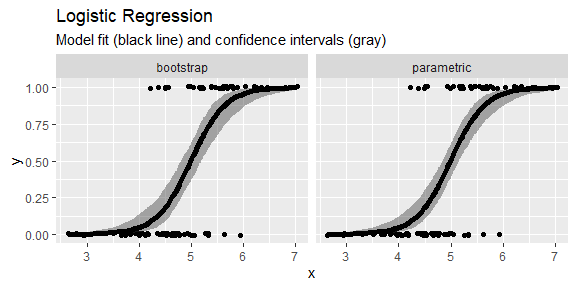Our two confidence interval methods mostly agree, but the bootstrap method produces slightly wider intervals.

## New names:
## * x -> x...1
## * y -> y...2
## * pred -> pred...3
## * type -> type...6
## * x -> x...7
## * ...

Another perspective on the difference between these two interval calculation methods is shown below. It’s a fairly clear that the BCa intervals (red) indeed exhibit little bias, but are not as tight as the parametric intervals (purple). This is expected behavior because the bootstrap confidence intervals don’t “know” about the model assumptions. In practice, if the sample size is small or the model is false, these interval estimates may exhibit more disagreement.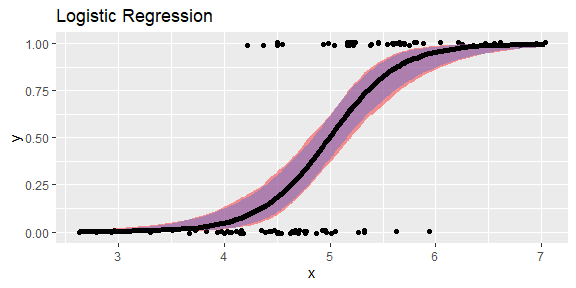If the sample size increases, we will find that the two estimates increasingly agree and converge to $$0$$ in width. Note that we do not calculate prediction intervals for $$y$$ when $$y$$ is Bernoulli distributed because the support of $$y$$ is exactly $$\{0,1\}$$.

## Prediction Intervals

Generally, parametric prediction intervals for GLMs are not available. The solution ciTools takes is to perform a parametric bootstrap on the model fit, then take quantiles on the bootstrapped data produced for each observation. The procedure is performed via arm::sim. The method of the parametric bootstrap is described by the following algorithm:

1. Fit the GLM, and collect the regression statistics $$\hat{\beta}$$ and $$\hat{\mathrm{Cov}}(\hat{\beta})$$. Set the number of simulations, $$M$$.

2. Simulate $$M$$ draws of the regression coefficients, $$\hat{\beta}_{*}$$, from $$N(\hat{\beta}, \hat{\mathrm{Cov}}(\hat{\beta}))$$, where $$\hat{\mathrm{Cov}}(\hat{\beta}) = \hat{\sigma}^2 (X'X)^{-1}$$.

3. Simulate $$[y_{*}|x]$$ from the response distribution with mean $$g^{-1}(x \hat{\beta}_{*})$$ and a variance determined by the response distribution.

4. Determine the $$\alpha/2$$ and $$1-\alpha/2$$ quantiles of the simulated response $$[y_{*}|x]$$ for each $$x$$.

The parametric bootstrap method propagates the uncertainty in the regression effects $$\hat{\beta}$$ into the simulated draws from the predictive distribution.

Generally, there are many different ways to calculate the quantiles of an empirical distribution, but the approach that ciTools takes ensures that estimated quantiles lie in the support set of the response. The choice we make corresponds to setting type = 1 in quantile().

We have seen in our simulations that the parametric bootstrap provides interval estimates with approximately nominal probability coverage. The unfortunate side effect of opting to construct prediction intervals through a parametric bootstrap is that the parameters of the predictive distributions need to hard coded for each model. For this reason, ciTools does not have complete coverage of all models one could fit with glm.

One exception to this scheme are GLMs with Gaussian errors. In this case we may parametrically calculate prediction intervals. Under Gaussian errors, glm() permits the use of the link functions “identity”, “log”, and “inverse”. The corresponding models are given by the following expressions:

$\begin{equation} \label{eq:gauss-link} \begin{split} y = X\beta + \epsilon\\ y = \exp(X\beta) + \epsilon \\ y = \frac{1}{X\beta} + \epsilon \end{split} \end{equation}$

The conditional response distribution in each case may be written $$y \sim N(g^{-1}(X\beta), \sigma^2)$$, and prediction intervals may be computed parametrically. Parametric prediction intervals are therefore constructed via

$\begin{equation} \label{eq:gauss-pi} g^{-1}(x'\beta) \pm t_{1-\alpha/2, n-p-1} \sqrt{\hat{\sigma}^2 + \hat{\sigma}^2x'(X'X)^{-1}x} \end{equation}$

for Gaussian GLMs. As in the linear model, $$\hat{\sigma}^2$$ estimates the predictive uncertainty and $$\hat{\sigma}^2 x(X'X)^{-1}x$$ estimates the inferential uncertainty in the fitted values. Note that $$g^{-1}(X \hat{\beta})$$ is a maximum likelihood estimate for the parameter $$g^{-1}(X \beta)$$, by the functional invariance of maximum likelihood estimation.

At this point, the only GLMs that we support with add_pi() are Guassian, Poisson, Binomial, Gamma, and Quasipoisson (all of which need to be fit with glm()). The models not supported by add_pi.glm are inverse Gaussian, Quasi, and Quasi-binomial.

## Poisson Example

Poisson regression is usually the first line of defense against count data, so we wish to present a complete example of quantifying uncertainty for this type of model with ciTools. For simplicity we fit a model on fake data.

We use rnorm to generate a covariate, but the randomness of $$x$$ has no bearing on the model.

x <- rnorm(100, mean = 0)
y <- rpois(n = 100, lambda = exp(1.5 + 0.5*x))
df <- data.frame(x = x, y = y)
fit <- glm(y ~ x , family = poisson(link = "log"))

As seen previously, the commands in ciTools are “pipeable”. Here, we compute confidence and prediction intervals for a model fit at the $$90\%$$ level. The warning message only serves to remind the user that precise quantiles cannot be formed for non-continuous distributions.

df_ints <- df %>%
add_ci(fit, names = c("lcb", "ucb"), alpha = 0.1) %>%
add_pi(fit, names = c("lpb", "upb"), alpha = 0.1, nSims = 20000) %>%
head()
## Warning in add_pi.glm(., fit, names = c("lpb", "upb"), alpha = 0.1, nSims =
## 20000): The response is not continuous, so Prediction Intervals are approximate

As with other methods available in ciTools the requested statistics are computed, appended to the data frame, and returned to the user as a data frame.

df_ints %>%
ggplot(aes(x = x, y = y)) +
geom_point(size = 2) +
ggtitle("Poisson Regression", subtitle = "Model fit (black line), with prediction intervals (gray), confidence intervals (dark gray)") +
geom_line(aes(x = x, y = pred), size = 1.2) +
geom_ribbon(aes(ymin = lcb, ymax = ucb), alpha = 0.4) +
geom_ribbon(aes(ymin = lpb, ymax = upb), alpha = 0.2)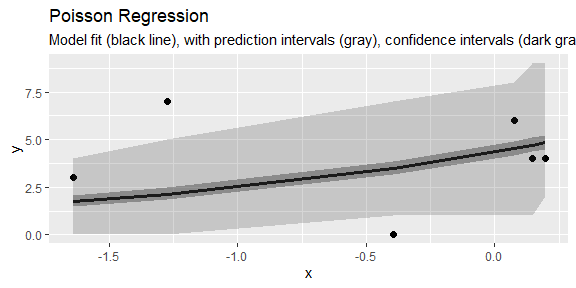Since the response $$y$$ is count data, and the method we used to determine the intervals involves simulation, we find that ciTools will produce “jagged” bounds when all the intervals are plotted simultaneously. Increasing the number of simulations using the nSims argument in add_pi can help reduce some of this unsightliness.

We may also wish to compute response-level probabilities and quantiles. ciTools can also handle these estimates with add_probs() and add_quantile() respectively. We use the same parametric bootstrap approach for estimating quantiles and probabilities that we employed for add_pi(). Once again, an error message reminds the user that their support is not continuous.

df %>%
head()
## Warning in add_probs.glm(., fit, q = 10): The response is not continuous, so
## estimated probabilities are only approximate
## Warning in add_quantile.glm(., fit, p = 0.4): The response is not continuous, so
## estimated quantiles are only approximate
##            x y     pred prob_less_than10 quantile0.4
## 1 -1.2725648 7 2.145159           0.9995           2
## 2 -0.3948852 0 3.492482           0.9965           3
## 3  0.1444020 4 4.711910           0.9755           4
## 4  0.0745726 6 4.532688           0.9780           4
## 5 -1.6400189 3 1.749197           1.0000           1
## 6  0.1952983 4 4.846988           0.9750           4

### Extension to Quasipoisson

A common problem with the Poisson model is the presence of over-dispersion. Recall that for the Poisson model, we require that the variance and mean agree, however this is practically a strict and unreasonable modeling assumption. A quasipoisson model is one remedy: it estimates an additional dispersion parameter and will provide a better fit. Under the quasipoisson assumption

$\mathbb{E}[y|X] = \mu = \exp (X \beta)$

and

$\mathbb{V}\mathrm{ar}[y|X] = \phi \mu$

Quasi models are not full maximum likelihood models, however it is possible to embed a Quasipoisson in the Negative Binomial framework using

$\mathrm{QP}(\mu, \theta) = \mathrm{NegBin}(\mu, \theta = \frac{\mu}{\phi - 1})$

Where NegBin is the parameterization of the Negative Binomial distribution used by glm.nb in the MASS library. This model for the negative binomial distribution, a continuous mixture of Poisson random variables with gamma distributed means, is preferred over that classical parameterization in applications. The preference stems from the fact that it allows for non-integer-valued $$\theta$$.

Warning: As in Gelman and Hill’s Data Analysis using Regression and Multilevel/Hierarchical Model, ciTools does not simulate the uncertainty in the over-dispersion parameter $$\hat{\phi}$$. According to our simulations, dropping this uncertainty from the parametric bootstrap has a negligible effect on the coverage probabilities. While the distribution of $$\hat{\phi}$$ is asymptotically Normal, it is very likely that the finite sample estimator has a skewed distribution. Approximating this distribution for use in a parametric bootstrap is ongoing research. As it stands, the prediction intervals we form for over-dispersed models tend to be conservative.

Negative binomial regression (via glm.nb) is implemented as a separate method in ciTools, and is an alternative to quasipoisson regression. For more information on the difference between these two models, we recommend Jay Ver Hoef and Peter Boveng’s Quasi-poisson vs. Negative Binomial Regression: How Should We Model Overdispersed Count Data?

### Example

Again, we generate fake data. The dispersion parameter is set to $$5$$ in the quasipoisson model.

x <- runif(n = 100, min = 0, max = 2)
mu <- exp(1 + x)
y <- rnegbin(n = 100, mu = mu, theta = mu/(5 - 1))

The data is over-dispersed:

df <- data.frame(x = x, y = y)
fit <- glm(y ~ x, family = quasipoisson(link = "log"))
summary(fit)\$dispersion
##  3.73578

But ciTools can still construct appropriate interval estimates for the range of a new observation:

df_ints <- add_ci(df, fit, names = c("lcb", "ucb"), alpha = 0.05) %>%
add_pi(fit, names = c("lpb", "upb"), alpha = 0.1, nSims = 20000)
## Warning in add_pi.glm(., fit, names = c("lpb", "upb"), alpha = 0.1, nSims =
## 20000): The response is not continuous, so Prediction Intervals are approximate
ggplot(df_ints, aes(x = x, y = y)) +
ggtitle("Quasipoisson Regression", subtitle = "Model fit (black line), with Prediction intervals (gray), Confidence intervals (dark gray)") +
geom_point(size = 2) +
geom_line(aes(x = x, y = pred), size = 1.2) +
geom_ribbon(aes(ymin = lcb, ymax = ucb), alpha = 0.4) +
geom_ribbon(aes(ymin = lpb, ymax = upb), alpha = 0.2)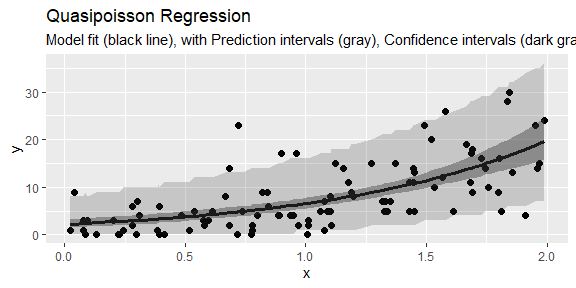The darker region represents the confidence intervals formed by add_ci and the lighter intervals are formed by add_pi. Again, intervals are “jagged” because the response the is not continuous and the bounds are formed through a simulation.

## Simulation Study for Prediction Intervals

A simulation study was performed to examine the empirical coverage probabilities of prediction intervals formed using the parametric bootstrap. We focus on these intervals because we could not find results in the literature addressing their performance. Our simulation is not comprehensive, so users of ciTools should exercise care when using these methods. In each simulation, a simple $$y = g^{-1}(mx + b)$$ model is fit on a variable number of observations.

New observations were generated from the true model to determine if the empirical coverage was close to the nominal level. The mean interval width was also recorded, as were standard errors of the estimated coverage and mean interval width.

Note that in contrast to a study of confidence intervals, we do not expect interval widths to shrink to $$0$$ as sample size tends to infinity. This is due to the predictive error in the conditional response distribution, which is not a factor in the construction of confidence intervals.

We take the same approach in the simulation study of each of the four models described below:

1. Set a sequence of sample sizes e.g. $$n = 20, 50, 100, ...$$.
2. For each sample size, set a model matrix
3. Then loop …
• Generate a response vector from the true model
• Fit a GLM to the simulated response $$\boldsymbol{y}$$ with the fixed model matrix
• Calculate a prediction interval for $$y_{new}$$ given $$x_0$$, the midpoint of the range of $$x$$ using $$2000$$ bootstrap replicates.
• Store the width of this prediction interval.
• Generate a response $$y_{new} | x_0$$ from the true model and determine if the new response is in the PI.
4. Repeat Step 3 $$M$$ times.

### Poisson

A Poisson model is fit with the log-link function.

$y|x \sim \mathrm{Poisson}(\lambda = \exp(1 + 2 x)), \quad x \in (1, 2)$

For each sample size, $$10,000$$ simulations were performed. We find that observed coverage levels are biased conservative by about $$0.5\%$$. This bias is likely a side effect of the type of quantile we calculate on the bootstrapped data. Interval widths generally decrease with sample size, however there is an increase from sample size $$500$$ to $$1000$$, which is within simulation error.

pois <- read.csv("pois_pi_results.csv") %>%
dplyr::select(-total_time) %>%
rename(nominal_level = level) %>%
dplyr::select(sample_size, everything())
knitr::kable(pois)
sample_size nominal_level cov_prob cov_prob_se int_width int_width_se
20 0.95 0.9585 0.0019945 28.9824 0.0089195
30 0.95 0.9568 0.0020332 28.9686 0.0083300
50 0.95 0.9611 0.0019337 28.9707 0.0079568
100 0.95 0.9547 0.0020797 28.9350 0.0077294
250 0.95 0.9573 0.0020219 28.9146 0.0073916
500 0.95 0.9567 0.0020354 28.9136 0.0074926
1000 0.95 0.9585 0.0019945 28.9161 0.0075347
2000 0.95 0.9542 0.0020906 28.8923 0.0075509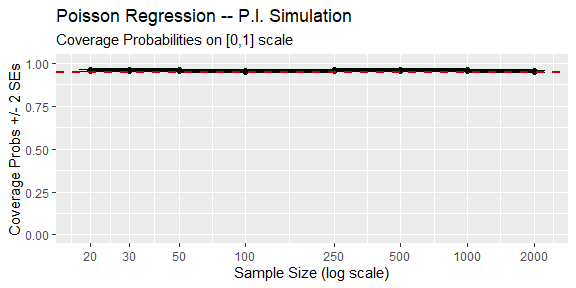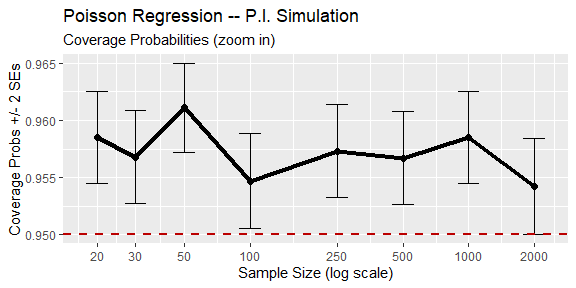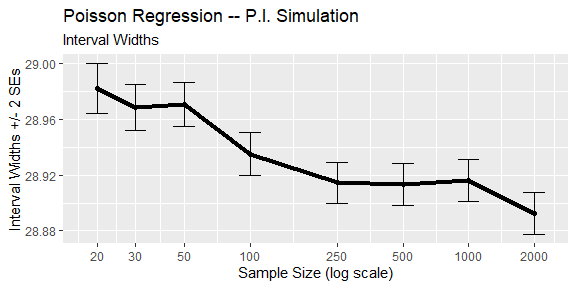### Negative Binomial

A negative binomial model with log link function was fit with dispersion parameter $$\theta = 4$$.

$y|x \sim \mathrm{NegBin}(\mu = \exp(1 + 2x), \theta = 4), \qquad x \in (1, 2)$

For each sample size, $$10,000$$ models were fit. Estimated coverage probabilities lie below the nominal level for small sample sizes. Statistical “folklore” recommends against fitting negative binomial models on a small sample, so our observations are in-line with this advice. Even though observed coverage probabilities closely agree with the nominal level, we find that interval width estimates tend to actually increase slightly with sample size, a trend we find concerning given the standard errors of the interval width estimates. Possible explanations of this trend could be our requirement that our prediction intervals are forced to lie in the positive integers, and that we do not simulate the distribution of the dispersion parameter. These results lead us to believe that more study is warranted on this type of interval estimate.

neg_bin <- read.csv("negbin_pi_results.csv") %>%
dplyr::select(-total_time) %>%
rename(nominal_level = level) %>%
dplyr::select(sample_size, everything())
knitr::kable(neg_bin)
sample_size nominal_level cov_prob cov_prob_se int_width int_width_se
20 0.95 0.9225 0.0026740 99.5669 0.1996143
30 0.95 0.9365 0.0024387 102.7880 0.1667759
50 0.95 0.9441 0.0022974 104.9926 0.1292217
100 0.95 0.9455 0.0022701 106.6165 0.0933470
150 0.95 0.9505 0.0021692 107.3229 0.0794959
200 0.95 0.9530 0.0021165 107.4567 0.0707095
250 0.95 0.9503 0.0021734 107.7405 0.0642865
500 0.95 0.9528 0.0021208 108.1632 0.0501491
1000 0.95 0.9560 0.0020511 108.1898 0.0407571
2000 0.95 0.9495 0.0021899 108.2864 0.0354954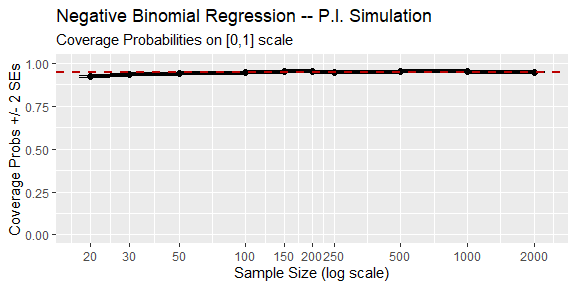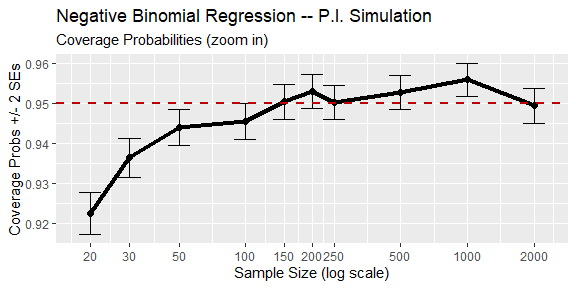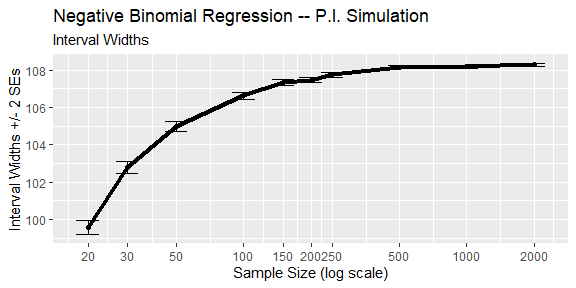### Gamma

A Gamma regression model with "inverse" link function was fit and simulated $$10,000$$ times.

$y|x \sim \Gamma(\mathrm{shape} = 5, \mathrm{rate} = \frac{5}{2 + 4x}) \qquad x \in (30, 70)$

Gamma regression was not discussed in this vignette but is still supported by ciTools. Estimated coverage probabilities are generally close, but consistently slightly below, the nominal level. In contrast to the results from the Poisson simulation, these coverage probabilities are within simulation error of the nominal level. Average interval widths tend to exhibit a high degree of variation.

gam <- read.csv("gamma_pi_results.csv") %>%
dplyr::select(-total_time) %>%
rename(nominal_level = level) %>%
dplyr::select(sample_size, everything())

knitr::kable(gam)
sample_size nominal_level cov_prob cov_prob_se int_width int_width_se
100 0.95 0.9466 0.0022484 343.8714 0.3424111
250 0.95 0.9492 0.0021960 339.1537 0.2634857
500 0.95 0.9468 0.0022444 348.9826 0.1639942
1000 0.95 0.9496 0.0021878 342.0288 0.1347365
2000 0.95 0.9463 0.0022544 346.6101 0.1121606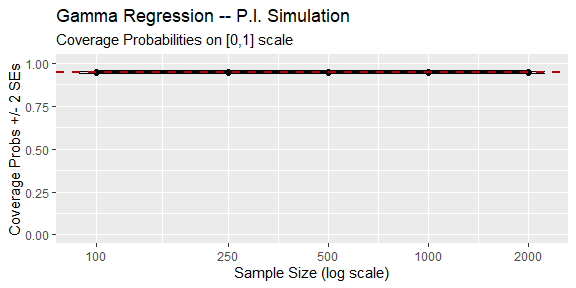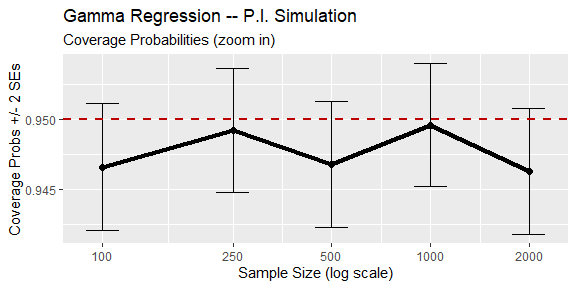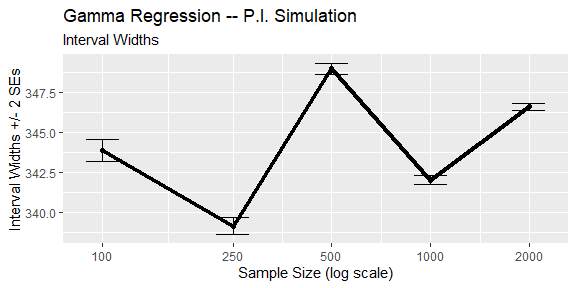In practice, the (non-canonical) log-link function is more common due to its numerical stability. Since the choice of link function does not drastically alter our prediction interval procedure, ciTools can also handle these types of models.

## Summary

ciTools is a versatile R package that helps users quantify uncertainty about their generalized linear models. Creating interval estimates that are amenable to plotting is now as simple as fitting a GLM. To date, we provide coverage for many common GLMs used by practitioners. For the models covered by ciTools, our simulations show that our confidence and prediction intervals are trustworthy.

There is still work to be done on this portion of ciTools. We would like to

1. Include more parametric methods for prediction intervals.

2. Add facilities to handle CIs and PIs when offset terms are present in the model fit.

3. Further study interval estimates pertaining to the negative binomial model.

4. Produce a simulation study that compares parametric vs. bootstrap intervals for GLMs.

5. Offer alternative prediction intervals e.g. the shortest intervals that contain $$95\%$$ of the simulated data.

6. Include options beyond BCa for creating bootstrap confidence intervals.

### Session

sessionInfo()
## R version 4.0.2 (2020-06-22)
## Platform: x86_64-w64-mingw32/x64 (64-bit)
## Running under: Windows 10 x64 (build 17763)
##
## Matrix products: default
##
## locale:
##  LC_COLLATE=C
##  LC_CTYPE=English_United States.1252
##  LC_MONETARY=English_United States.1252
##  LC_NUMERIC=C
##  LC_TIME=English_United States.1252
##
## attached base packages:
##  stats     graphics  grDevices utils     datasets  methods   base
##
## other attached packages:
##  arm_1.11-2    lme4_1.1-23   Matrix_1.2-18 MASS_7.3-51.6 knitr_1.29
##  ggplot2_3.3.2 ciTools_0.6.1 dplyr_1.0.1
##
## loaded via a namespace (and not attached):
##   statmod_1.4.34      tidyselect_1.1.0    xfun_0.16
##   purrr_0.3.4         splines_4.0.2       lattice_0.20-41
##   colorspace_1.4-1    vctrs_0.3.2         generics_0.0.2
##  htmltools_0.5.0     yaml_2.2.1          base64enc_0.1-3
##  utf8_1.1.4          survival_3.1-12     rlang_0.4.7
##  nloptr_1.2.2.2      pillar_1.4.6        withr_2.2.0
##  foreign_0.8-80      glue_1.4.2          RColorBrewer_1.1-2
##  jpeg_0.1-8.1        lifecycle_0.2.0     stringr_1.4.0
##  munsell_0.5.0       gtable_0.3.0        htmlwidgets_1.5.1
##  coda_0.19-3         evaluate_0.14       labeling_0.3
##  latticeExtra_0.6-29 fansi_0.4.1         highr_0.8
##  htmlTable_2.0.1     Rcpp_1.0.5          scales_1.1.1
##  backports_1.1.8     checkmate_2.0.0     Hmisc_4.4-1
##  abind_1.4-5         farver_2.0.3        gridExtra_2.3
##  png_0.1-7           digest_0.6.25       stringi_1.4.6
##  grid_4.0.2          cli_2.0.2           tools_4.0.2
##  magrittr_1.5        tibble_3.0.3        Formula_1.2-3
##  cluster_2.1.0       crayon_1.3.4        pkgconfig_2.0.3
##  ellipsis_0.3.1      data.table_1.13.0   assertthat_0.2.1
##  minqa_1.2.4         rmarkdown_2.3       rstudioapi_0.11
##  R6_2.4.1            boot_1.3-25         rpart_4.1-15
##  nnet_7.3-14         nlme_3.1-148        compiler_4.0.2